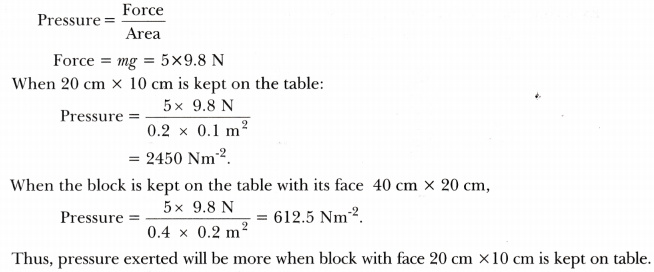# Explain, with the help of an example the difference between the terms thrust and pressure. Which one of the two has same SI unit as that of Forc

• Explain, with the help of an example the difference between the terms thrust and pressure. Which one of the two has same SI unit as that of Force?
• Consider a wooden block of mass 5 kg and dimensions 40 cm X 20 cm X 10 cm with its faces 20 cm X 10 cm and 40 cm X 20 cm kept on the table, in turn. In which case will the pressure exerted by the box on the table be more? Justify your answer by doing mathematical calculations.

•